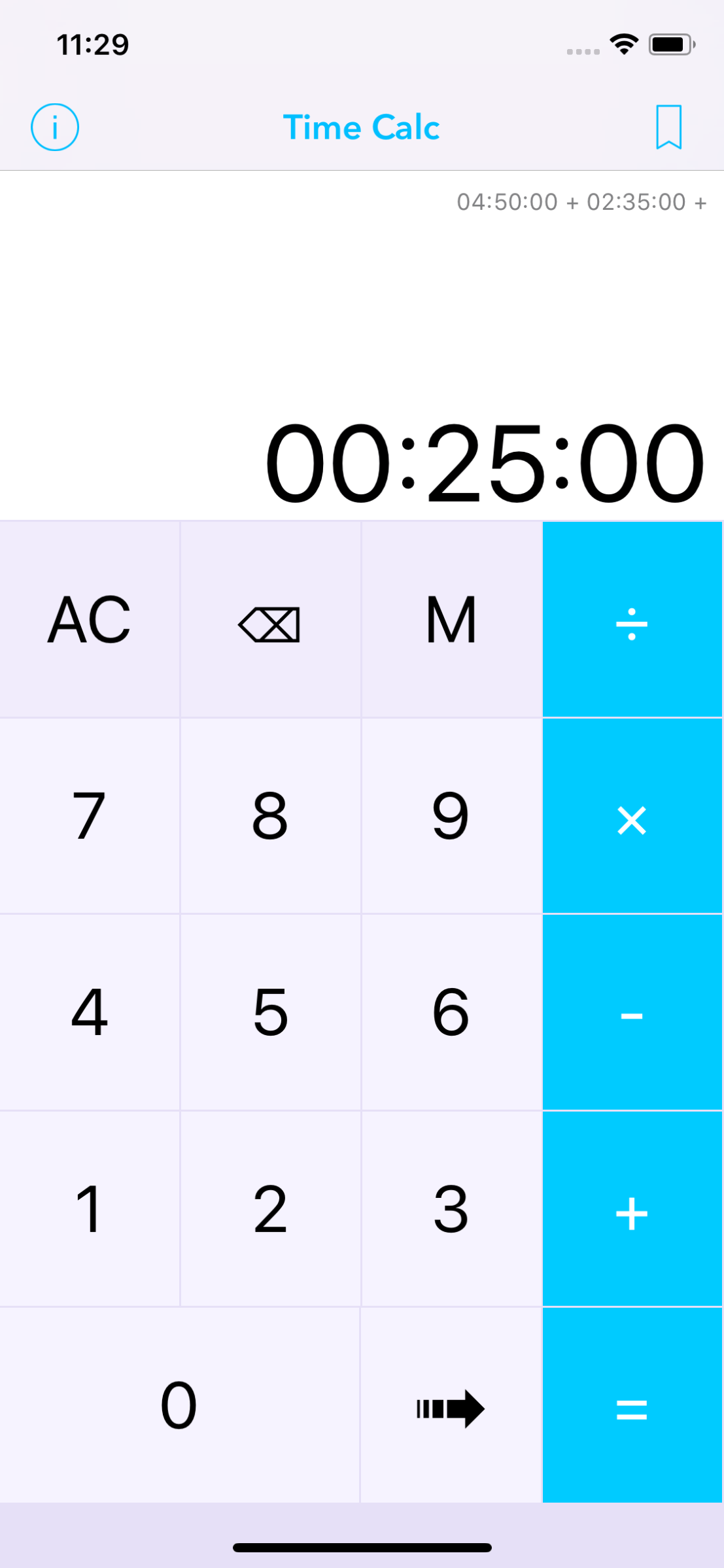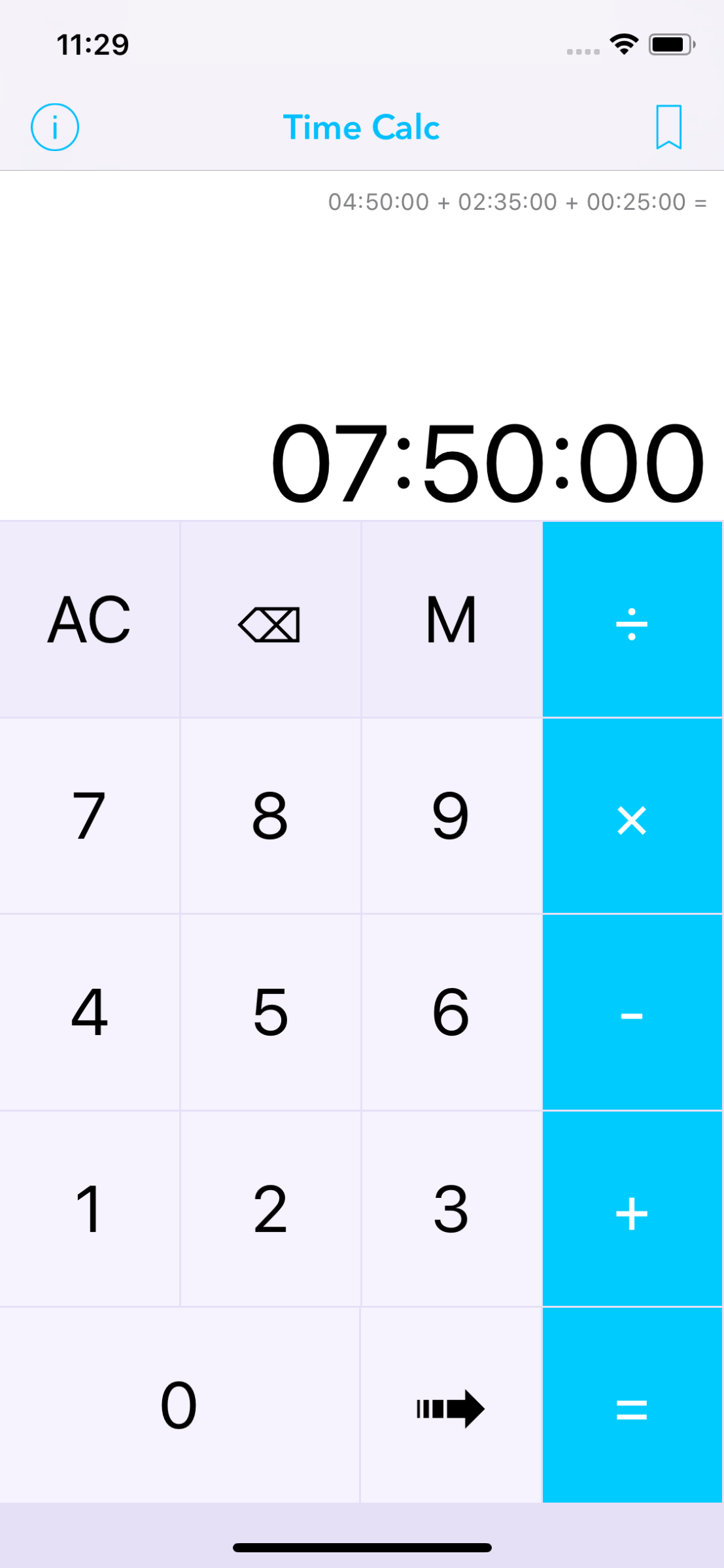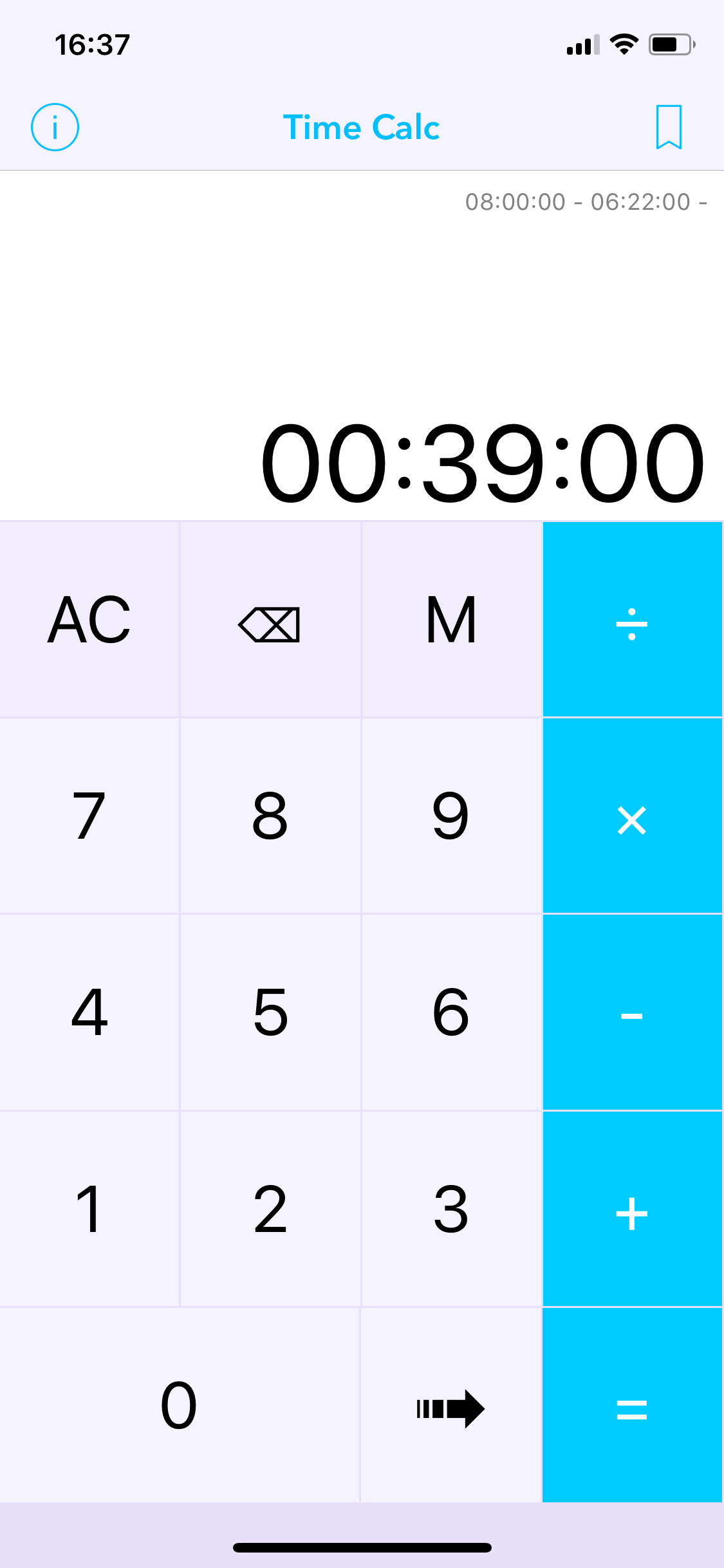# How to add or subtract time using Time Calc

Sometimes we need to perform elementary operations with time. Add hours or subtract minutes and seconds is not so easy. This is useful at work or if you need to log flight hours in a Logbook.

## Perform mathematical operations with time is difficult and only an application or excel are the fast and right way to calculate the result without errors.

Suppose you need to add two different time values together to get a total time spent working on a project.

As you’ll see in the sections below, Time Calc for Apple iPhone/iPad makes it easy to add or subtract time.## How to add hours and minutes using Time Calc.

Let’s say that you want to know how many hours and minutes it takes to complete three tasks. You estimate that the first task will take 4 hours and 50 minutes, the second task will take 2 hours and 35 minutes and the third task will take 25 minutes. Open Time Calc and insert: 4:50:00 press + and insert: 2:35:00 press + and insert: 00:25:00 and press = to get the result of  7 hours and 50 minutes## How to subtract hours and minutes using Time Calc.

Now you want to know how many hours and minutes it remains to complete your job after you have done two tasks. You estimate to work 8 hours, the first task has taken 6 hours and 22 minutes and the second task has taken 39 minutes. Open Time Calc and insert: 8:00:00 press – and insert: 6:22:00 press – and insert: 00:39:00 and press = to get the result of 59 minutes.### These are just some of the features you can find using Time Calc Pro:

• Calculate time in a simple and accurate way.
• Perform elementary operations (add, subtract, multiply and divide) on hours, minutes and seconds.
• Use a beautiful and elegant user interface.
• Convert the result to other units of time.
• Save and share important operations.
• Work in portrait or landscape mode.